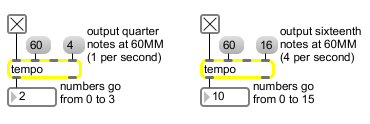# tempo

Output numbers at a metronomic tempo

## Description

tempo acts as a metronome controllable in beats per minute with specifiable whole-note divisions.

## Arguments

Name Type Opt Description
initial-tempo (5 through 300) int or float opt The first argument sets an initial tempo, from 5 to 300 beats per minute. If there is no argument, the initial tempo is 120 beats per minute. The second argument is the beat multiplier and is set to 1 by default. The third argument sets an initial rhythmic value of the output, from a whole note (1) to a 64th note triplet (96). If the argument is not present, the initial value is 16.

## Messages

 bang In left inlet: Starts the tempo object's metronome process, or restarts it if tempo is already on. int various (see description) [int] Converted to float. float various (see description) [float] In left inlet: If the number is not 0, it has the same effect as bang. If the number is 0, it has the same effect as stop. In 2nd inlet: The number is stored as the tempo, in beats per minute (quarter notes per minute). In 3rd inlet: The number is a beat multiplier, which can lengthen the amount of time taken for one beat. It slows the tempo down by a factor. For example, a multiplier of 2 will make tempo send out its output half as fast. In right inlet: The number is the rhythmic value sent out by tempo, specified as a fraction of a whole note. For example, the number 8 causes tempo to output eighth notes, relative to the specified (quarter note) tempo. The numbers sent out the outlet cycle continuously between 0 and the number 1 less than the rhythmic value. The divisions of a whole note must be between 1 and 96. clock setclock object name [symbol] The word clock, followed by the name of an existing setclock object, sets the tempo object to be controlled by that setclock object rather than by Max’s internal millisecond clock. The word clock by itself sets the tempo object back to using Max’s regular millisecond clock. stop In left inlet: Stops tempo. tempo current-tempo [list] In left inlet: The word tempo, followed by a float, sets the current tempo to the number.

## Output

int: When tempo is started it outputs numbers in a continuous cycle from 0 to the number 1 less than the specified rhythmic value. The speed at which the numbers are sent out is determined by the tempo (quarter note beats per minute) and the rhythmic value of the output (fraction of a whole note).

## ExamplesThe tempo (60) defines the speed of a quarter note division defines the pulse to be sent out